Next: Polynomials and polynomial functions Up: Pre-requisites Previous: Introduction of co-ordinates

# Conic sections

In the co-ordinate plane one can study more general geometric figures that those desribed by lines. In this section we undertake a rigorous study of conic sections. In particular, we find geometric criteria that distinguish the different conics. We also establish Steiner's construction of conic sections as the locus of intersection of a pair of rotating lines.

The first equation that is more complicated that the equation of a line as given above is one of the form

ax2 + bxy + cy2 + dx + ey + f = 0

where a, b and c are not all zero (in which case the equation would become that of a line). The locus of points (x, y) that satisfy this equation is called a conic or a conic section.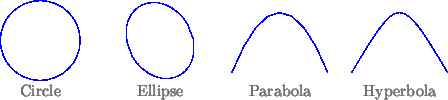By plotting the corresponding curves we find that we have the following types of conics:
1. There are no solutions.
2. The solutions all lie on one line.
3. The solutions all lie on a pair of lines.
4. The conic lies within a bounded region of the plane (i. e. the conic is compact). This called an ellipse (of which the circle is a special case).
5. The conic has two parts (i. e. the conic is disconnected).This is called a hyperbola.
6. The conic is connected and not compact. This is called a parabola.
We note that the first three types are distinguished without reference to order among numbers (or separation axioms in geometry) and so make sense over other fields. We will see below how we can distinguish the other conics in a purely algebraic way.

Exercise 5   Find ways of distinguishing the different conics by looking at the equation. (Hint: Examine the discriminant b2 - 4ac).

For any line ax + by + c = 0 with a non-zero, we can write the solutions in terms of one parameter as (-bt-c/a, t); similarly when b is not zero. We can also solve'' a conic. Let us suppose that the conic is not of type (1), (2) or (3) above. Fix a point (x0, y0) on the conic.

Exercise 6   We will find a parametric solution of a conic. (Hint: Use translation and scaling of co-ordinates to simplify the equations wherever possible).
1. Let (y - y0) = t(x - x0) be a line through this point. Show that there is at most one other point of the conic that lines on this line.
2. Find the co-ordinates of this point in terms of the constants a, b, c, d, e, f, x0, y0 and the parameter t.
3. Show that this parametric solution is not well defined at two values of t for a hyperbola.
4. Show that this parametric solution misses one point in the case of an ellipse or circle but is well-defined at all values of t.
5. Show that this parametric solution is not well defined for one value of t and misses one point or is well defined and misses no points on a parabola.

This can be carried further through Steiner's construction as follows. Let (x1, y1) another point on the conic.

Exercise 7   Show that there are constants A, B, C and D so that for any point (x2, y2) of the conic we have

s =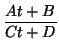whent =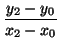ands =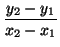Moreover, these constants are such that if we try to solve for s = t, we obtain no solutions when the conic is an ellipse (or circle), one solution for a parabola and two solutions for a hyperbola.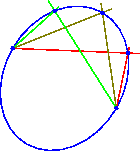The geometric content of this is the statement that the conic is obtained as the locus of intersection of a pair of rotating lines based at (x0, y0) and (x1, y1) respectively with respective slopes s and t related by s(Ct + D) = At + D.

Exercise 8   Prove the converse that such a locus is always a conic.Next: Polynomials and polynomial functions Up: Pre-requisites Previous: Introduction of co-ordinates
Kapil H. Paranjape 2001-01-20